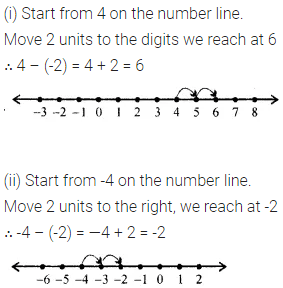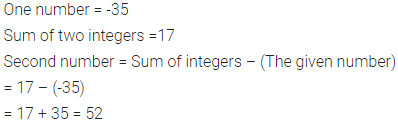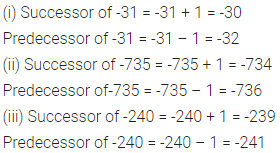# ML Aggarwal Class 6 Solutions for ICSE Maths Chapter 3 Integers Ex 3.3

## ML Aggarwal Class 6 Solutions for ICSE Maths Chapter 3 Integers Ex 3.3

Question 1.
Evaluate the following, using the number line:
(i) 4 – (-2)
(ii) -4 – (-2)
(iii) 3 – 6
(iv) -3 – (-5)
Solution:Question 2.
Subtract :
(i) -6 from 9
(ii) 6 from -9
(iii) -6 from -9
(iv) -725 from -63
(v) -376 from 10
(vi) 92 from -620
Solution:Question 3.
Evaluate the following:
(i) -237 – (+ 1884)
(ii) -346 – (- 1275)
(iii) -190 – (-3512)
(iv) -2718 – (+ 6827)
Solution:Question 4.
The sum of two integers is 17. If one of them is -35, find the other.
Solution:Question 5.
What must be added to -23 to get -9?
Solution:Question 6.
Find the predecessor of 0.
Solution:Question 7.
Find the successor and the predecessor of the following integers:
(i) -31
(ii) -735
(iii) -240
Solution: